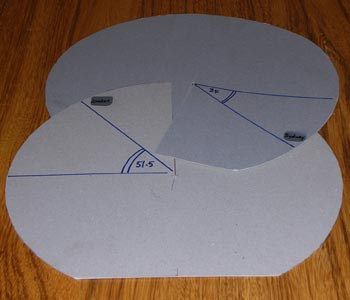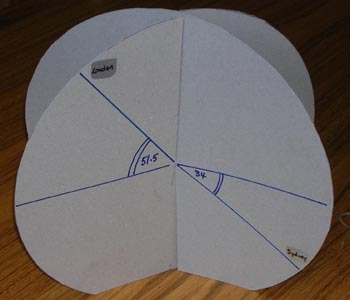#### You may also like### 2D-3D

Two circles of equal size intersect and the centre of each circle is on the circumference of the other. What is the area of the intersection? Now imagine that the diagram represents two spheres of equal volume with the centre of each sphere on the surface of the other. What is the volume of intersection?### The Dodecahedron Explained

What is the shortest distance through the middle of a dodecahedron between the centres of two opposite faces?### When the Angles of a Triangle Don't Add up to 180 Degrees

This article outlines the underlying axioms of spherical geometry giving a simple proof that the sum of the angles of a triangle on the surface of a unit sphere is equal to pi plus the area of the triangle.

# Flight Path

##### Age 16 to 18 Challenge Level:A model to demonstrate latitude and longitude Cut 2 discs of cardboard. Cut a radial slit in each so that they slot together as shown. Mark your chosen cities and their angles of latitude. In the illustration London at $51.5^o$ North and Sydney at $34^o$ South are shown. Cut off a small segment at the South Pole so that the model stands up. Adjust the angle between the two planes in the model to show the difference in their angles of longitude.Take the origin at the centre of the earth, the x-axis through the Greenwich Meridian at the equator, the y-axis through longitude $90^o$ East at the equator and the z-axis through the North Pole. London is approximately on the Greenwich Meridian so the 3-D coordinates of London are $(R\cos 51.5^o, 0, R\sin 51.5^o$).

As an extension to this question you might like to calculate the distance between Cape Town ($18^o$E, $34^o$S) and Sydney ($151^o$E, $34^o$S) along the great circle and find how much shorter it is than the distance around the line of latitude $34^o$S.